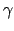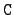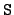Next: The I_number identifier of Up: Various identifiers Previous: The C Reaction Identifiers   Contents

# The S Reaction Switch

The S switch is one of the parameters in the structure nhead in the directory of the `library' file, and its purpose is the tell the significance of some other parameters in nhead, namely, X1, X2, X3, and X4. For an explanation of nhead see Section 3.2.3.

0:
There is no X data.

1:
The parameter X1 is the excitation level in Mev.

2:
These are pre-equilibrium and unresolved direct-interaction processes, and there is no X data. Note for example, that the ENDL library has two separate 235U(n,) reactions (= 46), a cluster reaction with= 0 and a direct-interaction process with= 2.

3:
We have gamma-ray production, and X1 is the energy of the emitted photon in Mev.

5:
This is an activation process, X1 = 1000*Z + A to identify the atomic number and mass number of the residual, X2 is the excitation level of the residual in Mev, and X3 is its halflife in seconds.

7:
We have delayed fission groups, and X1 is the halflife of this group. But if X1 = 0, then the data is a sum over all delayed groups.

8:
This is a time-sequential cluster model, and X1 gives the excitation level in Mev.

91:
In this case X1 designates the subshell.Next: The I_number identifier of Up: Various identifiers Previous: The C Reaction Identifiers   Contents